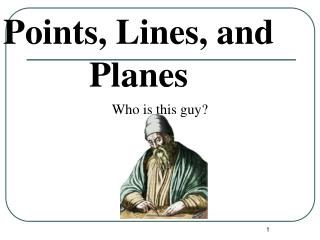DownloadDownload PresentationPoints, Lines, and Planes

# Points, Lines, and Planes

Télécharger la présentation## Points, Lines, and Planes

- - - - - - - - - - - - - - - - - - - - - - - - - - - E N D - - - - - - - - - - - - - - - - - - - - - - - - - - -
##### Presentation Transcript

1. Points, Lines, and Planes Who is this guy?

2. Points • Points do not have actual size. • How to Sketch: Using dots • How to label: Use capital letters Never name two points with the same letter (in the same sketch). A B C

3. Lines • Lines extend to no end and have no thickness or width. • How to sketch : using arrows at both ends. • How to name: 2 ways (1) small script letter – line n (2) any two points on the line - • Never name a line using three points - n A B C

4. Collinear Points • Collinear points are points that lie on the same line. (The line does not have to be visible.) • A point lies on the line if the coordinates of the point satisfy the equation of the line. Ex: To find if A (1, 0) is collinear with the points on the line y = -3x + 3. Substitute x = 1 and y = 0 in the equation. 0 = -3 (1) + 3 0 = -3 + 3 0 = 0 The point A satisfies the equation, therefore the point is collinear with the points on the line. A B C Collinear C A B Non collinear

5. Try these on your own:

6. Planes • A plane is a flat surface that extends indefinitely in all directions. • How to sketch: Use a parallelogram (4 sided figure) or triangle • How to name: (1) Any 3 non collinear points in the plane - Plane: ABC/ ACB / BAC / BCA / CAB / CBA A B C Horizontal Plane

7. Different planes in a figure: A B Plane ABCD Plane EFGH Plane BCGF Plane ADHE Plane ABFE Plane CDHG Etc. D C E F H G

8. Other planes in the same figure: Any three non collinear points determine a plane! Plane AFGD Plane ACGE Plane ACH Plane AGF Plane BDG Etc.

9. Coplanar Objects Coplanar objects (points, lines, etc.) are objects that lie on the same plane. The plane does not have to be visible. Are the following points coplanar? A, B, C ? Yes A, B, C, F ? No H, G, F, E ? Yes E, H, C, B ? Yes A, G, F ? Yes C, B, F, H ? No

10. Try these on your own:

11. Intersection of Figures The intersection of two figures is the set of points that are common in both figures. The intersection of two lines is a point. m Line m and line n intersect at point P. P n Continued…….

12. 2 Possibilities of Intersection of a Line and a Plane (1) Line passes through plane – intersection is a point. (2) Line lies on the plane - intersection is a line. (3) Line is parallel to the plane - no common points.

13. Intersection of Two Planes is a Line. B P A R Plane P and Plane R intersect at the line

14. Postulates and Axioms (1 of 2) • A postulate, or axiom is an accepted statement of fact.

15. Postulates and Axioms (2 of 2)